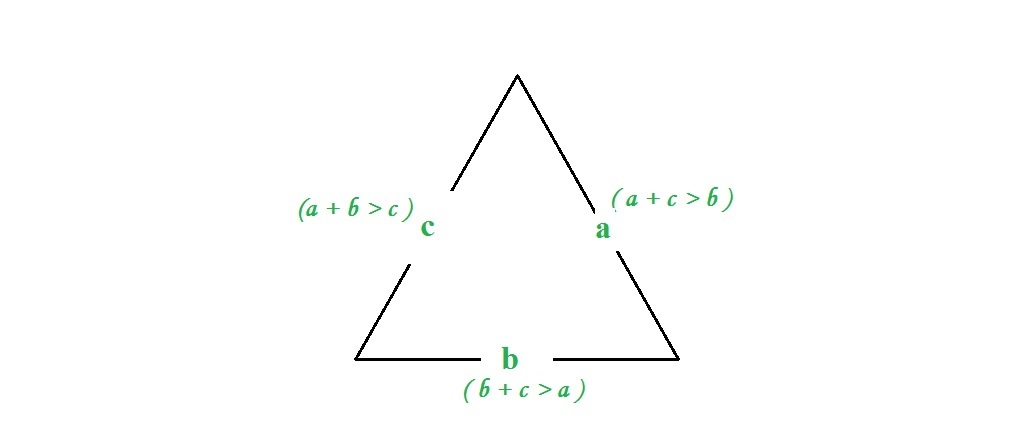# Check whether triangle is valid or not if sides are given

• Difficulty Level : Basic
• Last Updated : 07 Nov, 2021

Given three sides, check whether triangle is valid or not.
Examples:

```Input :  a = 7, b = 10, c = 5
Output : Valid

Input : a = 1 b = 10 c = 12
Output : Invalid```

Attention reader! All those who say programming isn't for kids, just haven't met the right mentors yet. Join the  Demo Class for First Step to Coding Coursespecifically designed for students of class 8 to 12.

The students will get to learn more about the world of programming in these free classes which will definitely help them in making a wise career choice in the future.

Approach: A triangle is valid if sum of its two sides is greater than the third side. If three sides are a, b and c, then three conditions should be met.

```1.a + b > c
2.a + c > b
3.b + c > a  ```## C++

 `// C++ program to check if three``// sides form a triangle or not``#include``using` `namespace` `std;`` ` `// function to check if three sider``// form a triangle or not``bool` `checkValidity(``int` `a, ``int` `b, ``int` `c)``{``    ``// check condition``    ``if` `(a + b <= c || a + c <= b || b + c <= a)``        ``return` `false``;``    ``else``        ``return` `true``;``}`` ` `// Driver function``int` `main()``{``    ``int` `a = 7, b = 10, c = 5;``     ` `    ``if` `(checkValidity(a, b, c))``        ``cout << ``"Valid"``;``    ``else``        ``cout << ``"Invalid"``;    ``}`

## Java

 `// Java program to check ``// validity of any triangle`` ` `public` `class` `GFG {`` ` ` ` `    ``// Function to calculate for validity``    ``public` `static` `int` `checkValidity(``int` `a,``                                ``int` `b, ``int` `c)``    ``{``        ``// check condition``        ``if` `(a + b <= c || a + c <= b || b + c <= a)``            ``return` `0``;``        ``else``            ``return` `1``;``    ``}`` ` `    ``// Driver function``    ``public` `static` `void` `main(String args[])``    ``{`` ` `        ``int` `a = ``7``, b = ``10``, c = ``5``;``     ` `        ``// function calling and print output``        ``if` `((checkValidity(a, b, c)) == ``1``)``            ``System.out.print(``"Valid"``);``        ``else``            ``System.out.print(``"Invalid"``);``         ` `    ``}``}`` ` `// This article is contributed by 'Akansh Gupta'`

## Python3

 `# Python3 program to check if three``# sides form a  triangle or not `` ` `# function to check if three sides ``# form a triangle or not ``def` `checkValidity(a, b, c): ``     ` `    ``# check condition ``    ``if` `(a ``+` `b <``=` `c) ``or` `(a ``+` `c <``=` `b) ``or` `(b ``+` `c <``=` `a) :``        ``return` `False``    ``else``:``        ``return` `True`        ` ` `# driver code ``a ``=` `7``b ``=` `10``c ``=` `5``if` `checkValidity(a, b, c):``    ``print``(``"Valid"``) ``else``:``    ``print``(``"Invalid"``)`

## C#

 `// C# program to check ``// validity of any triangle``using` `System;`` ` `class` `GFG {``     ` `    ``// Function to calculate for validity``    ``public` `static` `int` `checkValidity(``int` `a, ``int` `b, ``                                    ``int` `c)``    ``{``         ` `        ``// check condition``        ``if` `(a + b <= c || a + c <= b || ``                            ``b + c <= a)``            ``return` `0;``        ``else``            ``return` `1;``    ``}`` ` `    ``// Driver code``    ``public` `static` `void` `Main()``    ``{``        ``int` `a = 7, b = 10, c = 5;``     ` `        ``// function calling and print output``        ``if` `((checkValidity(a, b, c)) == 1)``          ``Console.Write(``"Valid"``);``        ``else``          ``Console.Write(``"Invalid"``);``         ` `    ``}``}`` ` `// This code is contributed by Nitin Mittal.`

## PHP

 ``

## Javascript

 ``

Output :

`Valid`

My Personal Notes arrow_drop_up